• Shuffle
Toggle On
Toggle Off
• Alphabetize
Toggle On
Toggle Off
• Front First
Toggle On
Toggle Off
• Both Sides
Toggle On
Toggle Off
Toggle On
Toggle Off
Front

## Card Range To Study

throughPlay buttonPlay buttonProgress

1/35

Click to flip

Use LEFT and RIGHT arrow keys to navigate between flashcards;

Use UP and DOWN arrow keys to flip the card;

H to show hint;

 Central tendency a single value used to describe the center point of a data set Measures of central tendency mean, median, and mode Mean AKA average, is the most common measure of central tendency;add all values in a data set and divide the result by the number of observations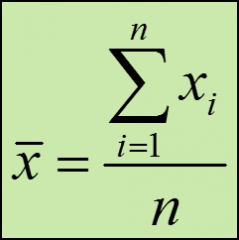x- bar = sample meanxi = the values in the samplen = the number of data values in the sample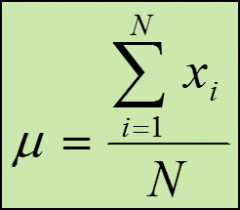Mu - the population meanN - the number of data values in the population Weighted mean allows you to assign more weight to certain values and less weight to others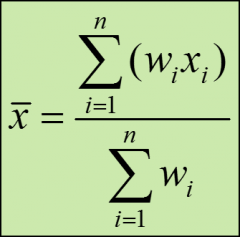wi - the weight for each data value xibottom of formula - the sum of all the weights Advantages of using mean to summarize data simple to calculatesummarizes the data with a single value disadvantages of using the mean to summarize data with only a summary value you lose information about the original data;the value of the mean is sensitive to outliers median the value in the data set for which half the observations are lower and half are higher;NOT sensitive to outliers formula for the index point for the median i = .5(n)whenever the index point isn't a whole number, round the value up to the next highest whole number mode value that appears most often in a data set;if no data value or category repeats, we say the mode doesn't exist;more than one mode can exist if 2 or more values tie for most frequent;particularly useful way to describe categorical data Which measure of central tendency should you use? mean is generally used, unless extreme outliers exist;if outliers are present, the median is often used, since the median isn't sensitive to outliers;for categorical data, the mode is the best choice Measures of variability show how much spread is present in the data;range, variance, and standard deviation range simplest measure of variation;difference between the highest value and the lowest value in a data set s^2 = sample variance Sample variance formula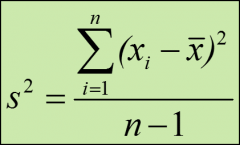(xi - xbar) = the difference between each data value and the sample mean Standard deviation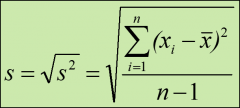the square root of the variance;has the same units at the original data Sample standard deviation formula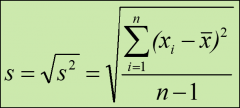Population variance formula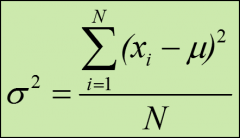coefficient of variation (CV) measures the standard deviation in terms of its percentage of the mean;a high cv indicates high variability relative to the size of the mean and vice-versa;a smaller coefficient of variation indicates more consistency within a set of data values sample coefficient of variation formula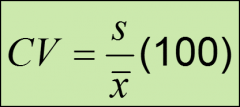population coefficient of variation formula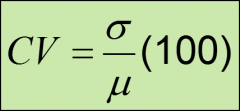z-score identifies the number of standard deviations a particular value is from the mean of its distribution;has no units;0 for values equal to the mean;positive for values above the mean;negative for values below the mean; z-score of an outlier is above +3 or below -3 population z-score formula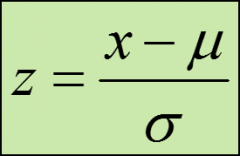sample z-score formula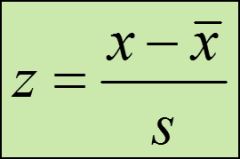The empirical rule if a distribution follows a bell-shaped, symmetrical curve centered around the mean, we would expect:approx 68% of the values to fall within +/- 1 standard deviations from the mean;approx 95% of the values to fall within +/- 2 standard deviations from the meanapprox 99.7% of the values to fall within +/- 3 standard deviations from the mean Measures of relative position Measures of relative position compare the position of one value in relation to other values in the data set;Percentiles and quartiles Percentiles measure the approx percentage of values in the data set that are below the value of interest find percentiles manually sort the data from lowest to highest;calculate the index point, i;if i is not a whole number, round it to the next whole number;if i is a whole number, the midpoint between i and i+1 position is our value percentile rank identifies the percentile of a particular value within a set of data percentile rank formula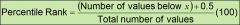quartiles split the ranked data into 4 equal groups:the 1st quartile (Q1) constitutes the 25th percentile;the 2nd quartile (Q2) constitutes the 50th percentile (also is the median);the 3rd quartile (Q3) constitutes the 75th percentile Interquartile Range (IQR) describes the middle 50% of a range;find the IQR by subtracting the first quartile from the third quartile;